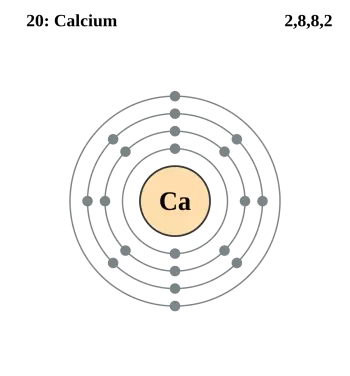# Atomic Mass of Calcium (ca)Calcium, a chemical element essential for life, is known for its role in the health of bones and teeth. However, the relevance of calcium goes beyond human biology and extends to a wide range of scientific fields.

The atomic mass of calcium is a physical and chemical concept that refers to the average mass of all calcium isotopes found in nature, weighted by their relative abundance.

Atomic mass is expressed in unified atomic mass units (uma) or daltons (Da), and for calcium, its average atomic mass is approximately 40,078 u.

## Calculation of the Atomic Mass of Calcium

The atomic mass of calcium is calculated by taking into account the relative abundance of its natural isotopes.

Calcium has several isotopes, the most abundant being calcium-40 (Ca-40), which represents approximately 96% of all natural calcium. Additionally, calcium-44 (Ca-44) is another common isotope, making up approximately 2.1% of natural calcium. Other isotopes of calcium, such as Ca-42 and Ca-48, are less abundant and have a minor effect on the average atomic mass.

The calculation of atomic mass is done by multiplying the mass of each isotope by its relative abundance and adding the results. In the case of calcium, this results in an average atomic mass of about 40,078 u.

### Table with the Atomic Masses of Calcium Isotopes

The following table shows the atomic mass of each calcium isotope and the percentage of its isotopic composition.

 Isotope Atomic mass (Da) Isotopic abundance (amount fraction) 40Ca  _ 39,962 5909 (2) 96.941% (156) 42  Ca 41,958 618 (1) 0.647% (23) 43  Ca 42,958 766 (2) 0.135% (10) 44  Ca 43,955 482 (2) 2.086% (110) 46  Ca 45,953 69 (2) 0.004% (3) 48  Ca 47,952 5229 (6) 0.187% (21)

Source CIAAW

## Properties and Characteristics of CalciumCalcium (Ca) is a chemical element of the periodic table with an atomic number of 20. This atomic number indicates that a calcium atom has 20 protons in its nucleus and, consequently, 20 electrons orbiting around it.

The pure form of calcium is a silvery-white alkaline earth metal, known for its reactivity and soft character. It was in 1808 that Sir Humphry Davy managed to isolate it in its pure form for the first time.

Due to its high chemical activity, calcium is not found freely in nature. However, it is possible to obtain pure metallic calcium through the process of electrolysis of a melt.

Calcium is the fifth most abundant element on Earth, after oxygen, silicon, aluminum and iron. Furthermore, it is an element found in large quantities in seawater, with an approximate concentration of 400 mg/l. In fact, it constitutes about 3.38% of the total mass of the Earth's crust.

This chemical element belongs to the group of alkaline earth metals and shares its high chemical activity with other members of this group of the periodic table. Despite their activity, there are other alkali metals that are even more reactive.

In nature, calcium is commonly found associated with carbon dioxide, oxygen and moisture present in the air, which contributes to its presence in various forms and compounds in our environment.

## Importance of the Atomic Mass of Calcium

The atomic mass of calcium is a concept of great importance in chemistry, physics and biology. This magnitude is not only essential for understanding the properties and behavior of calcium itself, but also has numerous applications in different scientific fields.

• In chemistry, the atomic mass of calcium is used to calculate the molar mass of calcium-containing compounds. Molar mass is crucial for formulating substances and understanding ratios in chemical reactions. For example, in calcium carbonate (CaCO₃), the molar mass is calculated by adding the mass of calcium, carbon and oxygen, which allows determining the amount of substance present in a sample.
• In nuclear physics, the atomic mass of calcium is essential to calculate the binding energy of atomic nuclei. Binding energy is necessary to understand processes such as nuclear fission and fusion, which are fundamental both in energy applications and in understanding the structure of matter.
• In astrophysics, the atomic mass of calcium is relevant in stellar nucleosynthesis, where heavier elements are formed from lighter elements inside stars. Calcium is produced in this process, and its atomic mass helps explain the abundance of calcium in the universe and how the elements are formed.
• In biology, calcium is essential for the health of living things, including humans. The atomic mass of calcium is key to calculating the amount of calcium needed in the diet and understanding its role in bone formation, nerve signal transmission, and many other biological functions.
Author:

Published: August 24, 2021
Last review: November 4, 2023